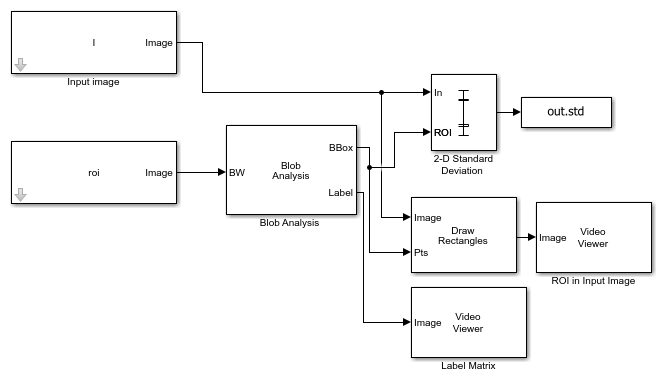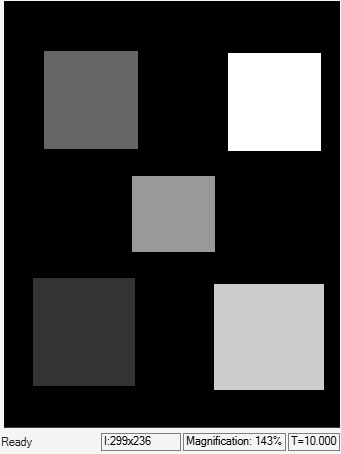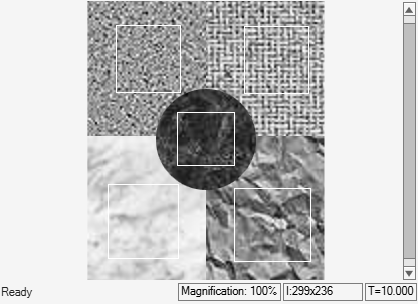Main Content

# Compute Standard Deviation of ROIs

This example shows how to compute the standard deviation of regions-of-interest (ROIs) in the input image. The input image is composed of different texture regions and ROIs are selected to contain these texture regions.

Read an image into the MATLAB workspace.

```I = imread('multitextures.png'); ```

Load the mask image that specifies the ROIs in the input image.

```load('binaryROI.mat') ```

### Example Model

Open the Simulink model.

```modelname='ex_blk2dstd.slx'; open_system(modelname); ```The model computes the coordinates for the ROIs by using the `Blob` `Analysis` block. The maximum number of blobs parameter in the `Blob` `Analysis` block is set to 5, the number of ROIs.

The `2-D` `Standard` `Deviation` block computes the standard deviation value for each ROI.

Set these parameters of the `2-D` `Standard` `Deviation` block to the specified value inorder to compute individual statistics for each ROI.

• Set `Find` `the` `standard` `deviation` `value` `over` parameter to `Entire` `input`

• Select `Enable` `ROI` `processing` parameter

• Set `ROI` `type` parameter to `Rectangles`

• Set `Output` parameter to `Individual` `statistics` `for` `each` `ROI`

### Simulate and Display Results

The values of the standard deviation indicate the dispersion of the pixel values in ROI from the corresponding mean value.

```out = sim(modelname); ```The model also displays the input image and the label matrix that correspond to the selected ROIs. The rectangles overlayed on the input image represents the ROIs for which the standard deviation is computed.

Display the standard deviation value for each ROI. The first standard deviation value correspond to the region with label value 1. Similarly, the second standard deviation value correspond to the region with label value 2 and so on.

```out.std ```
```ans = 0.0534 0.1203 0.0775 0.1463 0.1629 ```

Download ebook